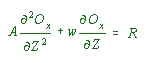REDOX ZONATION IN THE ANOXIC OCEAN

Pat WILDE
Pangloss Foundation
1735 Highland Place #28
Berkeley, California 94720
pat.wilde.td.57@aya.yale.edu

Mary S. QUINBY-HUNT
Lawrence Berkeley Laboratory
Berkeley, California 94720
mshunt@sbcglobal.net

ABSTRACT

Five principal redox zones in the open ocean: Oxic, Nitric, Sulfatic, Carbonic, and Nitronic are proposed based on the sequential thermodynamic availability of electron acceptors needed to oxidize marine planktonic organic matter. This is a chemical oceanographic refinement of the physical oceanographic model of redox conditions presented in Wilde . A sequence of such chemical zones may have been present in the ancient ocean when anoxic conditions were more common before the ventilation of the ocean with biogenically derived atmospheric oxygen and periodically since then, due to a combination of extended warm climatic intervals, poorer oceanic circulation and separation of basins from the global ocean. Maintenance of individual redox zones in the water column would occur in the pycnocline, where maximum stability and stratification are found. The redox boundaries in terms of equivalent oxygen for each zone is a function of the reservior of available oxidant and amount of reducing agent as planktonic organic matter and is calculated in terms of modern sea water chemistry. Generic formulae are given so that equivalent oxygen values can be calculated for varying oxidant reservoirs of the past. Equivalent oxygen values at the boundaries can be substituted into redox profiles with depth to obtain the thickness of each zone. The intersection of the various zones with the bottom would create different initial interstitial water chemistries for diagenetic water-sediment interactions, which may be discernible in the geologic record. An interactive computer model is presented generating a 2-D picture of the zones with inputs of % PAL and standard oceanic depths.

Calculator for demonstration purposes only. Still some bugs in deep calculations.

### OCEANIC VERTICAL REDOX CALCULATORafter Wilde (1987)

CLIMATE:ATMOSPHERE
Glacial
Pre-Post Glacial
Non Glacial
PAL%mL/L

Ts Depth DO mL/L
a
R0
A C1
C2
C3

D.O. at Specific Depth within the Pycnocline
DepthmetersDOmL/L

The model assumes that the oxidative decay proceeds (Riley, 1951; Wyrtki, 1962, p. 13) as:

R = Roe-aZ.............................. (1)
Where
R = rate of decay at depth Z
Ro = rate at the surface
a = function of oxidized substance
Z = depth

and the steady state concentration of oxygen (Ox) can be found by the solution of:......................................(2)
Where
A = vertical exchange coefficient

Wyrtki (1961, p. 44) has shown how the temperature profile can be used to calculate w/A from the expression:

wT - A T/z = wTD................... (3)

Where
T = potential temperature at given depth
TD= temperature at the depth of ascending water (base of the thermocline)

assuming, within the main thermocline, that (1) w is constant and (2) A is at a minimum. For the central part of the modern ocean he estimated that w = 2 x10-5 cm/sec and A = 0.5 cm2/sec were reasonable.

As noted in the text in eq (2) the concentration of oxygen can be modeled as:......................................(2)
Where
A = vertical exchange coefficient
R = RoeaZ

The solution of eq (2) for Os (oxidant) is:

Ox=C1 + C2e-(w/A)Z + C3e-aZ

where the constants of integration are:

C1 = Ox (@ upper boundary) - C2e-(w/A)Z (@upper boundary) - C3e-aZ(@ upper boundary);

C2 = [{Ox (@ upper boundary)--Ox (@ lower boundary)} -
{C3e-aZ (@ Upper boundary) +C3e-aZ (@ Lower boundary)} /
{e-(w/A)Z (@ Upper boundary) - e-(w/A)Z (@ Lower boundary)} ];

C3 = Ro/Aa(a - w/A)

Taken as constants are a = 0.000025 and Ro = 7.5 X 10-9.

Taken as constant for a particular climate (table 1) is the ratio (w/A), which is related to variation in temperature in the thermocline.